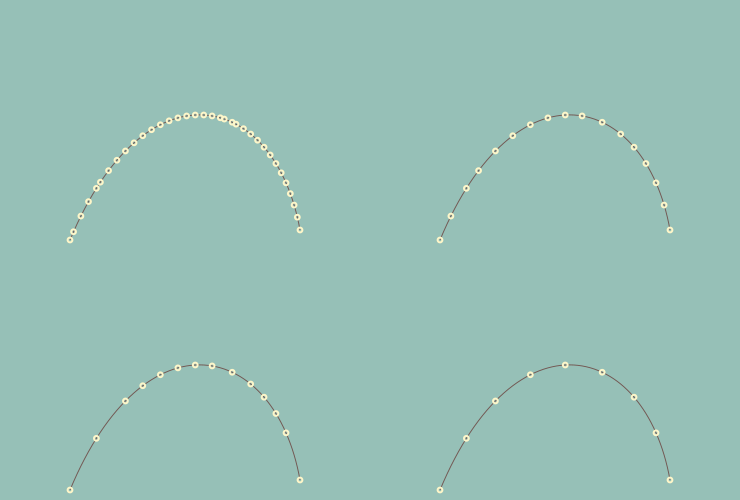# Crate points_on_curve

Expand description

This crate is a rustlang port of points-on-curve npm package written by @pshihn.

This package exposes functions to sample points on a bezier curve with certain tolerance. There is also a utility funtion to simplify the shape to use fewer points. This can really be useful when estimating lines/polygons for curves in WebGL or for Hit/Collision detections. Reverse of this operation is also supported meaning given some points generate bezier curve points passing through this points

### 📦 Cargo.toml

``````[dependencies]
points_on_curve = "0.1"
``````

### 🔧 Example

``````use euclid::{default, point2};
use points_on_curve::points_on_bezier_curves;

let input = vec![
point2(70.0, 240.0),
point2(145.0, 60.0),
point2(275.0, 90.0),
point2(300.0, 230.0),
];
let result_015 = points_on_bezier_curves(&input, 0.2, Some(0.15));``````

### 🖨️ Output

This picture shows computed points with 4 different distance values 0.15, 0.75, 1.5 and 3.0 with tolerance 2.0.### 🔭 Examples

For more examples have a look at the examples folder.

## Re-exports

`pub use euclid;`

## Functions

Generates Bezier Curve parameters passing through given points
computes distance squared from a point p to the line segment vw